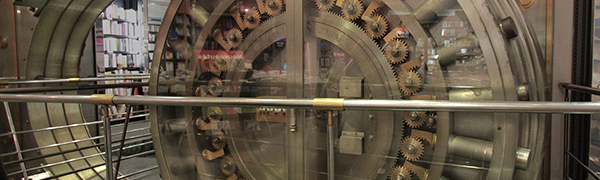# Decoding the code

Logic Level 2A thief entered a bank to rob it. Unfortunately, he forgot the actual code. However, he still remembers the following clues :-

1. All the digits are from 0-9, and each digit is used exactly once.
2. The sum of the 2nd, 5th, 6th and 8th digits is a single digit.
3. The 4th digit is a perfect square.
4. The 3rd digit is 1.
5. The sum of the 4th and the 7th digits is a perfect square.
6. The value of the 10th digit is twice that of the 2nd digit.
7. The product of the 4th and 6th digits is equal to the product of the 10th and 2nd digits.
8. The 5 is next to the 6, but not next to the 4.

What is the code?

Note: The 1st digit is the one on the extreme left. The 10th digit is the one on the extreme right.

×